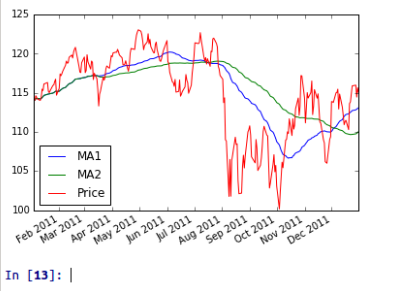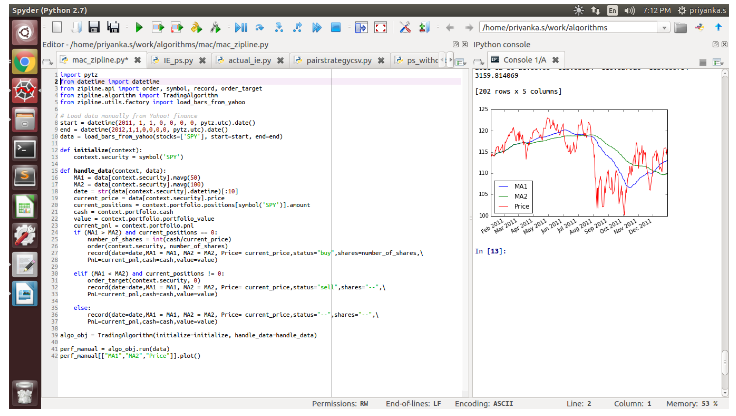# Zipline Python: Benefits, Installation, Structure and More

### Introduction

Python has emerged as one of the most popular languages for programmers in financial trading, due to its ease of availability, user-friendliness, and the presence of sufficient scientific libraries like Pandas, NumPy, PyAlgoTrade, Pybacktest and more.

Python serves as an excellent choice for automated trading when the trading frequency is low/medium, i.e. for trades which do not last less than a few seconds. It has multiple APIs/Libraries that can be linked to make it optimal, cheaper and allow greater exploratory development of multiple trade ideas.It is due to these reasons that Python has a very interactive online community of users, who share, reshare, and critically review each other’s work or codes. One of the most popular web-based backtesting systems is QuantConnect.

QuantConnect utilises C# and Python. It boasts of providing a wealth of historical data. QuantConnect has supported live trading with Interactive Brokers since 2015.

Zipline is a Python library for trading applications. It is an event-driven system that supports both backtesting and live trading.

In this article, we will learn how to install Zipline and then how to implement Moving Average Crossover strategy and calculate P&L, Portfolio value etc.

This article is divided into the following four sections:

• Benefits of Zipline
• Installation (how to install Zipline on local)
• Structure (format to write code in Zipline in Python),
• Coding Moving average crossover strategy with Zipline in Python.

### Benefits of Zipline

• Ease of use
• Zipline comes “batteries included” as many common statistics like moving average and linear regression can be readily accessed from within a user-written algorithm.
• Input of historical data and output of performance statistics are based on Pandas DataFrames to integrate nicely into the existing PyData ecosystem
• Statistic and machine learning libraries like matplotlib, scipy, statsmodels, and sklearn support development, analysis, and visualization of state-of-the-art trading systems

### Installation

#### Using pip

Assuming you have all required non-Python dependencies, you can install Zipline in Python with pip via:

# code
pip install Zipline

#### Using conda

Another way to install Zipline in Python is via the conda package manager, which comes as part of Anaconda or can be installed via pip install conda.

Once conda has been set up you can install Zipline from the conda-forge channel:

#code
conda install -c conda-forge zipline

### Basic structure

Zipline in Python provides a particular structure to the code which includes defining few functions that run the algorithms over a dataset as mentioned below.

#code
from Zipline.api import order, record, symbol
def initialize(context):
pass

def handle_data(context, data):
order(symbol('AAPL'), 10)
record(AAPL=data.current(symbol('AAPL'), 'price'))

perf_manual = algo_obj.run(data)

So, first we have to import some functions we would need in the code. Every Zipline algorithm consists of two functions you have to define:

* initialize(context) and * handle_data(context, data)

Before the start of the algorithm, Zipline calls the initialize() function and passes in a context variable. Context is a global variable that allows you to store variables you need to access from one algorithm iteration to the next.

After the algorithm has been initialized, Zipline calls the handle_data() function once for each event. At every call, it passes the same context variable and an event frame called data containing the current trading bar with open, high, low, and close (OHLC) prices as well as volume for each stock.

All functions commonly used in the algorithm can be found in Zipline.api module. Here we are using order(arg1, arg2) that takes two arguments: a security object, and a number specifying how many stocks you would like to order (if negative, order() will sell/short stocks). In this case, we want to order 10 shares of Apple at each iteration.

Now, the second method record() allows you to save the value of a variable at each iteration. You provide it with a name for the variable together with the variable itself. After the algorithm finished running you can all the variables you recorded, we will learn how to do that.

To run the algorithm, you would need to call TradingAlgorithm() that uses two arguments: initialize function and handle_data.  Then, call run method using data as an argument on which algorithm will run (data is panda data frame that stores the stocks prices)

run() first calls the initialize() function, and then streams the historical stock price day-by-day through handledata(). After each call to handledata() we instruct Zipline to order 10 stocks of AAPL.

### How to code Moving average crossover strategy with Zipline in Python

#### Moving Averages

It is the simple average of a security over a defined number of time periods.

#### Crossover

Moving average crossovers are a common way traders can use Moving Averages. A crossover occurs when a faster Moving Average (i.e. a shorter period Moving Average) crosses either above a slower Moving Average (i.e. a longer period Moving Average) which is considered a bullish crossover or below which is considered a bearish crossover.

Now we will learn how to implement this strategy using Zipline in Python. To import libraries and initialize variables that will be used in the algorithm.

The code is divided into 5 parts

• Initialization
• Initialize method
• handle_data method
• Strategy logic
• Run Algo

#### Initialization

#code

import pytz
from datetime import datetime
from Zipline.api import order, symbol, record, order_target
import pyexcel
# Load data manually from Yahoo! finance
start = datetime(2011, 1, 1, 0, 0, 0, 0, pytz.utc).date()
end = datetime(2012,1,1,0,0,0,0, pytz.utc).date()

data = load_bars_from_yahoo(stocks=['SPY'], start=start,end=end)

loadbarsfrom_yahoo() is the function that takes stock and time period for which you want to fetch the data. Here I am using SPY stocks between 2011 to 2012, you can change this according to you.

#### Initialize method

#code
def initialize(context):
context.security = symbol('SPY')

Now we would define initialize function, context.security represents the stock that we are dealing with, in our case its SPY.

#### handle_data method

#code
def handle_data(context, data):
MA1 = data[context.security].mavg(50)
MA2 = data[context.security].mavg(100)
date = str(data[context.security].datetime)[:10]
current_price = data[context.security].price
current_positions = context.portfolio.positions[symbol('SPY')].amount
cash = context.portfolio.cash
value = context.portfolio.portfolio_value
current_pnl = context.portfolio.pnl

handle_data() contains all the operation we want to do, the main code for our algorithm. we need to calculate moving averages for different windows, Zipline gives an inbuilt function mavg() that takes an integer to define the window size.

Also, Zipline automatically calculates currentprice, portfoliovalue etc. we can just call the variables, in this algorithm, I have calculated currentpositions, price, cash, portfoliovalue, and the PnL.

#### Strategy logic

#code (this will come under handle_data function only)
if (MA1 > MA2) and current_positions == 0:
number_of_shares = int(cash/current_price)
order(context.security, number_of_shares)
record(date=date,MA1 = MA1, MA2 = MA2, Price=

elif (MA1 < MA2) and current_positions != 0:
order_target(context.security, 0)
record(date=date,MA1 = MA1, MA2 = MA2, Price= current_price,status="sell",shares="--",PnL=current_pnl,cash=cash,value=value)

else:
record(date=date,MA1 = MA1, MA2 = MA2, Price= current_price,status="--",shares="--",PnL=current_pnl,cash=cash,value=value)

Now the logic that will place the order for buy or sell depending upon the condition that compares moving averages.

1. If short moving average is greater than longer one and your current_positions is 0 then you need to calculate the no of shares and place an order
2. If the short moving average is smaller than the longer one and your current_positions is not 0 then you need to sell all the shares that you have currently.
3. the third condition is if nothing satisfies then do nothing just record the variables you need to save.

#### Run

For running this algorithm, you need the following code:

#code
perf_manual = algo_obj.run(data)
perf_manual[["MA1","MA2","Price"]].plot()

You can plot the graph also using method plot()

#### Graph for the strategySnapshot of the screen using Zipline### Conclusion

We hope that you found this introduction to zipline in Python and implementing a strategy using the same useful. In our next article, we will show you how to import and backtest data in CSV format using Zipline. For building technical indicators using python, here are few examples.

### Next Step

Learn importing and backtesting on Zipline using data from Google and OHLC data in CSV format. Calculate backtesting results such as PnL, number of trades, etc in our post 'Importing CSV Data in Zipline for Backtesting'.

Update

We have noticed that some users are facing challenges while downloading the market data from Yahoo and Google Finance platforms. In case you are looking for an alternative source for market data, you can use Quandl for the same.

Disclaimer: All investments and trading in the stock market involve risk. Any decisions to place trades in the financial markets, including trading in stock or options or other financial instruments is a personal decision that should only be made after thorough research, including a personal risk and financial assessment and the engagement of professional assistance to the extent you believe necessary. The trading strategies or related information mentioned in this article is for informational purposes only.